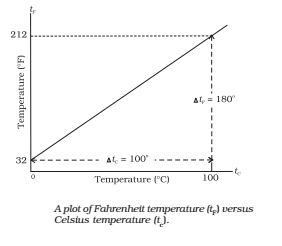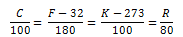The branch dealing with measurement of temperature is called thermometry and the devices used to measure temperature is called thermometers.

## Heat

Heat is a form of energy that flows between a body and its surrounding medium by virtue of temperature difference between them. The degree of hotness of the body is quantitatively represented by temperature.

Heat or thermal energy of a body is the sum of kinetic energies of all its constituent particles, on account of translational, vibration and rotational motion.

1. The SI unit of heat energy is joule (J).
2. The practical unit of heat energy is the calorie.
1 cal = 4.18 J
3. 1 calorie is the quantity of heat required to raise the temperature of 1 g of water by 1°C.
4. Mechanical energy or work (W) can be converted into heat (Q) by 1 W = JQ
where J = Joule’s mechanical equivalent of heat.
5. J is a conversion factor (not a physical quantity) and its value is 4.186 J/cal.

### Temperature

A temperature-measuring device (thermometer) makes use of some measurable property (called thermometric property) that change with temperature. Different thermometers lead to different temperature scales.

The highest possible temperature achieved in the laboratory is about 108 while lowest possible temperature attained is 10-8 K.

### Different Scale of Temperature:

• Celsius Scale: In this scale of temperature, the melting point ice is taken as 0°C and the boiling point of water as 100°C and space between these two points is divided into 100 equal parts.
• Fahrenheit Scale: In this scale of temperature, the melt point of ice is taken as 32°F and the boiling point of water as 211 and the space between these two points is divided into 180 equal parts.
• Kelvin scale: In this scale of temperature, the melting point ice is taken as 273 K and the boiling point of water as 373 K the space between these two points is divided into 100 equal parts.Relation between Different Scales of Temperatures### Differences between Heat and Temperature:

HeatTemperature
Heat is a form of energy which produces in us the sensation of hotness or coldness.Temperature is the degree of hotness or coldness of a body.
It is a cause when some heat is supplied to a body, its temperature increases.It is an effect.
It represents the total kinetic energy of the molecules of a body.It represents the average kinetic energy possessed by the molecules of a body.
Heat flows from high-temperature side irrespective of the amounts of heat possessed by the bodies in contact.Temperature decides the direction of flow of heat from one body to another.
It is measured in cal, kcal or joule.It is measured in ℃, ℉ or K.